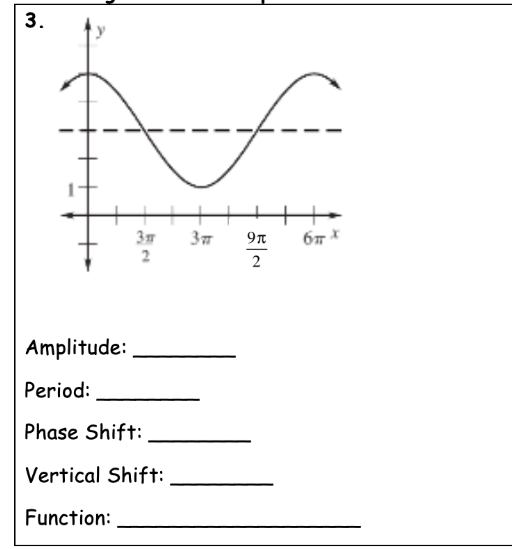# Examine the graph below and determine the amplitude, period, phase shift, and vertical shift ofeach using SINE as the parent function. Then write an equation of the function. 3.ofЗяЗтбтх9лAmplitude:Period:Phase Shift:Vertical Shift:Function:

Question
1 viewshelp_outlineImage TranscriptioncloseExamine the graph below and determine the amplitude, period, phase shift, and vertical shift of each using SINE as the parent function. Then write an equation of the function. fullscreenhelp_outlineImage Transcriptionclose3. of Зя Зт бтх 9л Amplitude: Period: Phase Shift: Vertical Shift: Function: fullscreen
check_circle

Step 1

Given graph is of sine which is a periodic function.

Amplitude is defined as the height from the center line to the peak or trough. It is obtained by finding the difference between the highest and lowest points and then dividi...

### Want to see the full answer?

See Solution

#### Want to see this answer and more?

Solutions are written by subject experts who are available 24/7. Questions are typically answered within 1 hour.*

See Solution
*Response times may vary by subject and question.
Tagged in